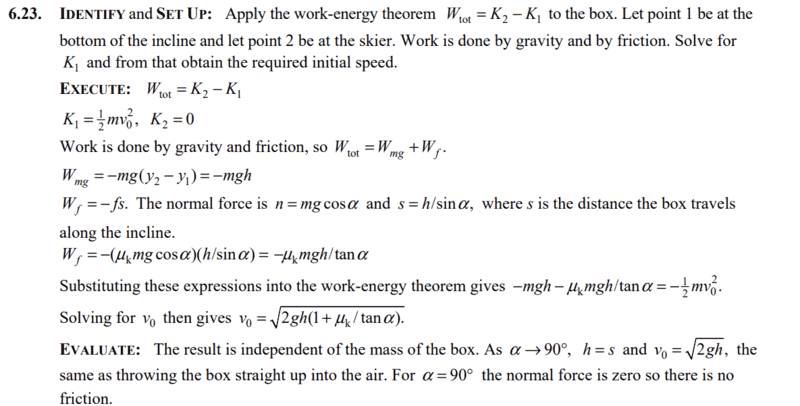# Work Done by Friction & Gravity on Incline: Explained

• simphys
In summary, the work done by gravity is equal to the displacement along the incline multiplied by the cosine of the angle between the incline and the horizontal direction.f

#### simphys

Homework Statement
You are a member of an Alpine Rescue Team. You must project
a box of supplies up an incline of constant slope angle a so that it reaches a
stranded skier who is a vertical distance h above the bottom of the incline.
The incline is slippery, but there is some friction present, with kinetic friction coefficient mk. Use the work–energy theorem to calculate the minimum speed you must give the box at the bottom of the incline so that it
will reach the skier. Express your answer in terms of g, h, mk, and a
Relevant Equations
##W = Fscos(alpha)##
where ##s=h/sin(alpha)##
So for the work done by the kinetic friction, the displacement along the incline is ##s## as given.
What I canNOT understand is why the displacement in the y-direction is used for the work done by gravity i.e. ##W = -mgh## where ##h## is the displacement in het y-direction. This instead of the displacement along the incline
This is complete bullshit, the work done on a force was supposed to be the component along the displacement wasn't it ?

Edit: this was the answer from the bookLast edited:
Using the displacement in the force direction and the full force magnitude is completely equivalent to using the force component in the displacement direction and the full displacement.

Using the displacement in the force direction and the full force magnitude is completely equivalent to using the force component in the displacement direction and the full displacement.
What a disaster... of course, thanks a lot! it comes down to the same thing but a little bit of a different formula.

The formula is the same, ##W = F d \cos\theta##, the difference is in the interpretation of that formula in words.

Last edited:
What a disaster... of course, thanks a lot! it comes down to the same thing but a little bit of a different formula.
Our incline of constant slope angle works as a simple machine: the inclined plane.
##W{gravity}~=~-mgh~=~-mgscos\alpha##

Copied from
https://en.wikipedia.org/wiki/Inclined_plane#Inclined_plane_with_friction

"Moving an object up an inclined plane requires less force than lifting it straight up, at a cost of an increase in the distance moved. The mechanical advantage of an inclined plane, the factor by which the force is reduced, is equal to the ratio of the length of the sloped surface to the height it spans. Owing to conservation of energy, the same amount of mechanical energy (work) is required to lift a given object by a given vertical distance, disregarding losses from friction, but the inclined plane allows the same work to be done with a smaller force exerted over a greater distance.
...
The mechanical advantage MA of a simple machine is defined as the ratio of the output force exerted on the load to the input force applied. For the inclined plane the output load force is just the gravitational force of the load object on the plane, its weight Fw. The input force is the force Fi exerted on the object, parallel to the plane, to move it up the plane."

I take it ##\alpha## is supposed to be the angle between the incline and the horizontal direction.

When you write ##W=Fs\cos\alpha##, what does ##\alpha## represent? Is it the same angle as above? If so, are you sure it's the right angle to be using when calculating the work?

The formula is the same, ##W = F d \cos\theta##, the difference is in the interpretation of that formula in words.
I prefer the interpretation that treats the vectors on an equal footing, "The work done by a constant force is the product of three quantities: (a) the magnitude of the force vector; (b) the magnitude of the displacement vector; (c) the cosine of the angle between the two."

What a disaster... of course, thanks a lot! it comes down to the same thing but a little bit of a different formula.
In fact for a conservative force (which includes gravity and other forces but not friction) the work done is path independent. The gravitational work calculated along any path from 1 to 2 will produce the same number.
So you can just choose a horizontal segment (Wgrav=0) plus a vertical segment (Wgrav=-mgh) Have you not seen this in your text?